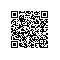# 位运算的威力

昨天写了篇有关集合的东西，中间用到了位运算，看起来还是有点纠结的，今天在这里详细解释一下。

（欢迎转载，转载请注明出处，谢谢。）

int a = 11;
int b = 1000;
(a)(00000000 00001011 )2                                            //a的二进制表示
(b)(00000011 11101000 )2                                            //b的二进制表示
a&b =(00000000 00001000 )=(8)10                             //一一为一，其它为0
a|b =  (00000011 11101011 )=(1003)10                    //有一为一，零零为0
a^b = (00000011 11100011 )=(995)10                       //相同为0，不相同为1
~b =   (11111100 00010111 )2 =(-31767)10               //按位取反
b>>3 =  (00000000 01111101 )2 =(125)10                   // 去掉低3位，高位补0

a<<3 =  (00000000 01011000 )2 =(88)10                    //去掉高3位，低位补0

位运算应用口诀

假如我是一个网管，公司内部使用C类地址，现在我要把公司网络划分成5个子网，网络号为192.168.1.0的前三段，那么子掩码怎么填呢？
我现在先告诉你子网的子网掩码分别怎么填：192.168.1.224。(当然这里还有其他答案，我取的是在子网扩充不超过8个的情况下的每个子网所容纳主机最多的最佳方案)。
这个怎么来的呢？ip本身是个二进制的东东，为了方便人们设置，我们采用了点分十进制的转换，把32位的ip地址转换成了4个字节的十进制莱表示。比如 192.168.1.213 这个ip地址的二进制表示为：11000000 10101000 00000001 11010101 。对于C类地址默认的前三个字节表示网络号，那么这个网络号就是：11000000 10101000 00000001   ，最后一个字节11010101表示主机号，可以知道这个网络可以容纳的最多主机数为2^8-2，为什么减2自己去查。现在要划分子网，那么我们就要从表示主机的那个字节也就是8个位里面拿出几个位来表示子网号， 几位比较合适呢？这就要看你需要划分多少个子网咯。比如我们现在要划分5个子网，(5)10 = (101)2 ，那么至少就需要3位了，而且最多可以划分2^3 = 8个子网。现在你把224换成二进制看看吧(224)10 = (11100000)2  ,明白了吧，我们可以推断出子网掩码干了什么勾当？不错子网掩码与ip地址做了按位与运算，他的作用就是屏蔽了主机号获取网络号与子网号。如果你明白了这点，你就知道自己在192.168.1.64子网的ip该怎么填了，不会错误滴填成192.168.1.10了。
竟然扯到一边去了，讲了半天才讲了一个与运算的应用。

int x = 32760;int y = 32762; 要求求x、y的平均值，要求空间复杂度位O(0)。
你能用常规方法去解决吗？可以。我不会讲，这里只讲位运算的 方法。
int ave(int x, int y)   //返回X、Y的平均值
{
return (x & y) + ( (x^y)>>1 );
}

x,y对应位均为1，相加后再除以2还是原来的数，如两个00001000相加后除以2仍得00001000,那么我们把x与y分别分成两个部分来看,两者相同的位分别拿出来 则 :
x = (111111111111000)2 =  (111111111111000)2 +  (000000000000000)2
y =  (111111111111010) (111111111111000)2 +  (000000000000010)2

int insert(BitSet* s,int index){
if(index >=0 && index>>3 < s->size)
{s->array[index>>3] |= (1<< (index & 7) );return 1}
return 0;
}

以上是我自己的一些学习心得。下面将贴上一些网络上的例子。
应用举例
(1) 判断int型变量a是奇数还是偶数
a&1   = 0 偶数
a&1 =   1 奇数
(2) 取int型变量a的第k位 (k=0,1,2……sizeof(int))，即a>>k&1   (先右移再与1)

(3) 将int型变量a的第k位清0，即a=a&~(1<<k)    (10000 取反后为00001 )

(4) 将int型变量a的第k位置1，即a=a|(1<<k)

(5) int型变量循环左移k次，即a=a<<k|a>>16-k   (设sizeof(int)=16)
(6) int型变量a循环右移k次，即a=a>>k|a<<16-k   (设sizeof(int)=16)

(7)对于一个数 x >= 0，判断是不是2的幂。

boolean power2(int x)
{
return ( (x&(x-1))==0) && (x!=0);
}

(8)不用temp交换两个整数
void swap(int x , int y)
{
x ^= y;
y ^= x;
x ^= y;
}

(9)计算绝对值

int abs( int x )
{
int y ;
y = x >> 31 ;
return (x^y)-y ;        //or: (x+y)^y
}

(10)取模运算转化成位运算 (在不产生溢出的情况下)
a % (2^n) 等价于 a & (2^n - 1)
(11)乘法运算转化成位运算 (在不产生溢出的情况下)
a * (2^n) 等价于 a<< n
(12)除法运算转化成位运算 (在不产生溢出的情况下)
a / (2^n) 等价于 a>> n
例: 12/8 == 12>>3
(13) a % 2 等价于 a & 1
(14) if (x == a)

x= b;
else      x= a;
等价于 x= a ^ b ^ x;
(15) x 的 相反数 表示为 (~x+1)
(16)输入2的n次方：1 << 19
(17)乘除2的倍数：千万不要用乘除法，非常拖效率。只要知道左移1位就是乘以2，右移1位就是除以2就行了。比如要算25 * 4，用25 << 2就好啦

功能              |          示例            |    位运算
----------------------+---------------------------+--------------------使用钉钉扫一扫加入圈子
+ 订阅